High School Math : Limits

Example Questions

Example Question #1 : Understanding The Definition Of Limits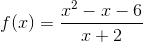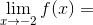Explanation:

A limit describes what-value a function approaches asapproaches a certain value (in this case,). The easiest way to find what-value a function approaches is to substitute the-value into the equation.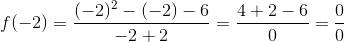Substitutingforgives us an undefined value (which is NOT the same thing as 0). This means the function is not defined at that point. However, just because a function is undefined at a point doesn't mean it doesn't have a limit. The limit is simply whichever value the function is getting close to.

One method of finding the limit is to try and simplify the equation as much as possible: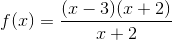As you can see, there are common factors between the numerator and the denominator that can be canceled out. (Remember, when you cancel out a factor from a rational equation, it means that the function has a hole -- an undefined point -- where that factor equals zero.)

After canceling out the common factors, we're left with: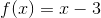Even though the domain of the original function is restricted (cannot equal), we can still substitute into this simplified equation to find the limit at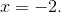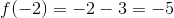Example Question #1 : Limits

Let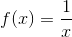.

Find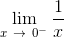.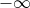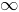The limit does not exist.Explanation: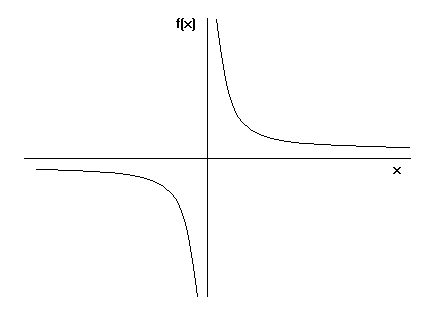This is a graph of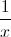. We know that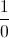is undefined; therefore, there is no value for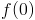. But as we take a look at the graph, we can see that asapproaches 0 from the left,approaches negative infinity.

This can be illustrated by thinking of small negative numbers.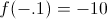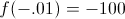NOTE: Pay attention to one-sided limit specifications, as it is easy to pick the wrong answer choice if you're not careful.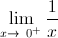is actually infinity, not negative infinity.

Example Question #2 : Limits

Evaluate the limit below: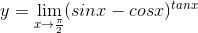0

1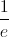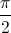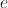Explanation:will approach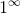whenapproaches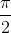, so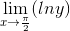will be of type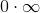as shown below: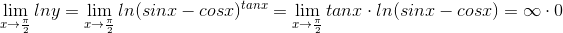So, we can apply the L’ Hospital's Rule: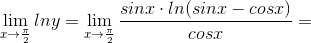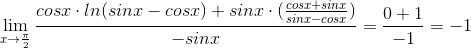since: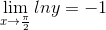hence: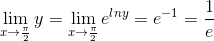Example Question #1 : Finding Limits As X Approaches Infinity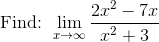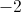Explanation: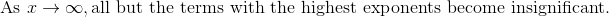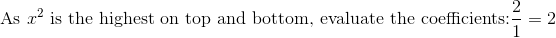Example Question #1 : Finding Limits As X Approaches Infinity

Calculate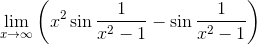.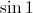The limit does not exist.Explanation:

This can be rewritten as follows: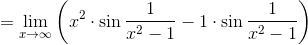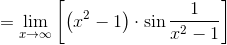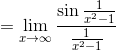We can substitute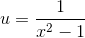, noting that as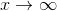,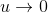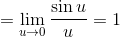, which is the correct choice.

Example Question #2 : Limits

The speed of a car traveling on the highway is given by the following function of time: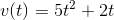What can you say about the car's speed after a long time (that is, asapproaches infinity)?

Nothing can be concluded from the given function.

The speed of the car depends on the starting speed.

The speed of the car approaches infinity.

The speed of the car approaches a constant number.

The speed of the car approaches zero.

The function given is a polynomial with a term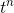, such thatis greater than 1.
Whenever this is the case, we can say that the whole function diverges (approaches infinity) in the limit asapproaches infinity.
This tells us that the given function is not a very realistic description of a car's speed for large!